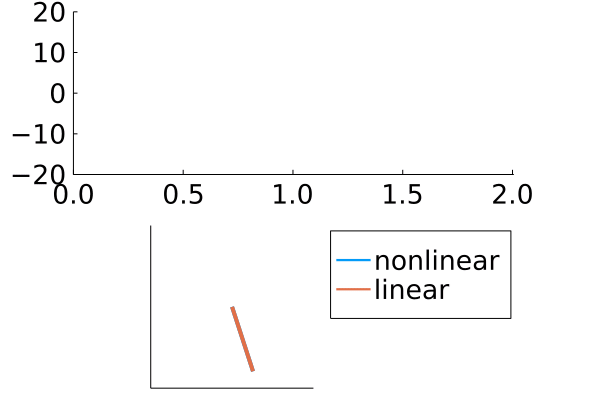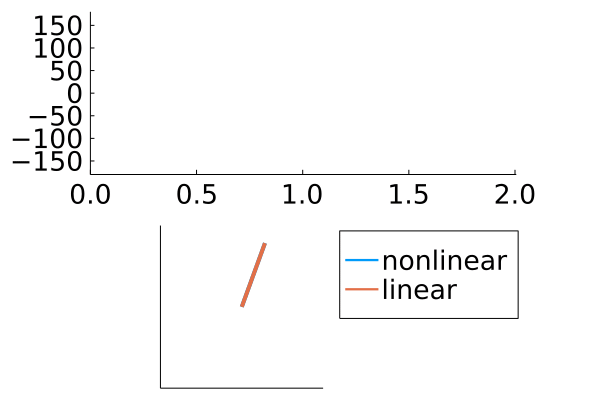# Day_05: Building a pendulum solution (linear and nonlinear)¶

Today, I wanted to build a Julia solution that I work on with students: comparing the small-angle approximation to the numerical integration for large angles in a simple pendulum. When a pendulum swings, there are two forces acting on the mass: tension in the cord, $$T$$, and gravity $$mg$$. The general equation of motion is

$$\ddot{\theta} + \frac{g}{r}\sin\theta = 0$$

where $$g$$ is the acceleration due to gravity, $$r$$ is the pendulum length, $$\theta$$ is the angle of the pendulum, and $$\ddot{\theta}$$ is the angular acceleration of the pendulum. As an engineer, I love to linearize these problems to get rid of that $$\sin$$-function. The function becomes linear by taking the Taylor series

$$\sin\theta = \theta - \frac{\theta^3}{3!} + \frac{\theta^5}{5!} + ...$$

and truncating to just

$$\sin\theta \approx \theta$$.

Now, the linear equation of motion is

$$\ddot{\theta} + \frac{g}{r}\theta = 0$$

The solution to this second order differential equation is that

$$\theta(t) = A\cos\omega t + B\sin\omega t$$

where $$A$$ and $$B$$ are integration constants solved with the initial angle and angular velocity and $$\omega = \sqrt{\frac{g}{r}}$$ is the natural frequency of the pendulum.

I have a linear second order differential equation with an exact solution and a nonlinear second order differential equation with no exact solution. Now, I’m ready to integrate these differential equations.

using DifferentialEquations, Plots, LaTeXStrings


## Plotting the linear solution for small angles¶

The first step is to plot the linear solution for small angles, when $$\sin\theta \approx \theta$$. I activate my Plots.theme(:cooper) which looks much better now with everything up-to-date.

I create my variables

• $$g$$ is g = 9.81 m/s/s

• $$r$$ is r = 0.25 m

• $$\omega$$ is w = sqrt(g/r) in rad/s

• $$t$$ is t = range(0, 4*pi/w, length = 200) is the time defined over two period of oscillation ($$2\pi~rad/cycle$$)

• $$\theta(t)$$ is a_lin = pi/10*cos.(w*t)

• This is solution for $$\theta(t)$$ when the initial speed is 0 and initial angle is $$\frac{\pi}{10}~rad=18^o$$

• The cos.(w*t) takes the cosine of each value of w*t. Without the . you get a MethodError

Plots.theme(:cooper)

g = 9.81
r = 0.25
w = sqrt(g/r)
t = range(0, 4*pi/w, length = 200)
a_lin = pi/10*cos.(w*t)

plot(t, a_lin*180/pi, xlabel = "time (s)", ylabel = L"$\theta$ (deg)")## Define the nonlinear differential equation¶

Solving a nonlinear differential equation requires a function that takes the current state, $$\theta~and~\dot{\theta}$$ and returns the change in the state, $$\dot{\theta},~\ddot{\theta}$$. In this pendulum function,

• u is the state variable $$[\theta,~\dot{\theta}]$$

• params are the differential equation parameters, in this case just pendulum length, $$r$$

• t is the current time. In this natural solution, the change in state is constant.

The pendulum function returns the change in state

du = [u, -g/r*sin(u)] = $$\left[\begin{array}{c} \frac{d\theta}{dt}\\ \frac{d\dot{\theta}}{dt} \end{array}\right] = \left[\begin{array}{c} \dot{\theta}\\ -\frac{g}{r}\sin\theta \end{array}\right]$$

function pendulum(u, params, t)
du = zeros(length(u))
r = params
du = u
du = -g/r*sin(u)
return du
end

pendulum (generic function with 1 method)


Checking the pendulum function, if $$\theta = 90^o=\frac{\pi}{2}$$, then the acceleration should be $$-g/r = -39~rad/s/s$$.

pendulum([pi/2, 0], [0.25], 0)

2-element Vector{Float64}:
0.0
-39.24


## Solve the nonlinear differential equation¶

The DifferentialEquations solutions takes two steps:

1. define the ODEProblem which includes

• the differential equations, pendulum

• intitial conditions [pi/10, 0]

• timespan, tspan

• parameters, p=r

2. solve the problem to create the DifferentialEquations solution object, sol

tspan = (0, 4*pi/w);
p = r;
prob = ODEProblem(pendulum, [pi/10, 0], tspan, p)
sol = solve(prob)

retcode: Success
Interpolation: specialized 4th order "free" interpolation, specialized 2nd order "free" stiffness-aware interpolation
t: 25-element Vector{Float64}:
0.0
8.220693010679318e-5
0.000904276231174725
0.009124969241854042
0.031792388774681435
0.06832437919393072
0.1151475767039386
0.1757149418556407
0.2470938476011576
0.32324737850646845
0.4070495658296888
0.506472739194497
0.6066783347570622
0.7135568815378345
0.8310676992969676
0.9400129569786826
1.0682386294892527
1.1813179664312168
1.3149043636733424
1.434280567197709
1.5700070508528805
1.6921528193982343
1.8307202855162528
1.9536541040753408
2.006066680710647
u: 25-element Vector{Vector{Float64}}:
[0.3141592653589793, 0.0]
[0.31415922438593635, -0.0009968269592066795]
[0.3141543076332868, -0.010965041242477706]
[0.3136545672025335, -0.1105904985847719]
[0.3080503858263155, -0.38308851330852756]
[0.28626549819935293, -0.8045789268217272]
[0.23704759523094662, -1.2831341323229977]
[0.14443524947953004, -1.7389362411909244]
[0.01022847992654069, -1.9588207308917562]
[-0.134495447492881, -1.7698418273919712]
[-0.25812716215303366, -1.1140106366851543]
[-0.3141384346740413, 0.0223958367072965]
[-0.25304117066526105, 1.1584199230014585]
[-0.08397657855890955, 1.8880019133926662]
[0.14013590253023944, 1.7526677083313713]
[0.28553501965400674, 0.814620146598685]
[0.2932874892203824, -0.7000447114220708]
[0.15071547920849154, -1.7180343819613828]
[-0.10266060148827366, -1.8515779674830302]
[-0.276543646528494, -0.9271892400931975]
[-0.29524014675307536, 0.6678588788213823]
[-0.13989677019501998, 1.7535787849149718]
[0.12317456412452218, 1.8020759480536648]
[0.28907885279879897, 0.7653044289196647]
[0.3132717359505, 0.1499375982817004]


## Compare small angle solutions linear vs nonlinear¶

Now, I have the linear $$\theta(t)$$ solution, a_lin and the _nonlinear solution sol.

Note: I got a little tripped up here. sol(0.1) will return an interpolated value for both $$\theta(0.1)$$ and $$\dot{\theta}(0.1)$$, but if I ask for sol(t) where t is the 200-element Vector I get a new object with both sol.t: time and sol.u:state. The object, sol.u is a Vector{Float64} so I can’t call out a single column e.g. sol.u is $$\theta(0)$$ and $$\dot{\theta}(0)$$. It felt strange after years of avoiding for-loops in NumPy and MATLAB, I made a list comprehension to grab the angles at each interpolated time.

[sol(ti) for ti in ti = t]


Its Julia, so I shouldn’t have to vectorize my work, but I just can’t help it.

First step, is to just compare angle, $$\theta$$ vs time.

a_nlin = [sol(ti) for ti = t]
plot(t, a_nlin*180/pi, label = "nonlinear")
plot!(t, a_lin*180/pi, label = "linear",
xlabel = "time (s)",
ylabel = L"$\theta$ (deg)")This looks great, the time periods and amplitudes match up almost exactly. To look at a more refined view, I can subtract the two angles and check the error

a_nlin = [sol(ti) for ti = t]
plot(t, (a_nlin - a_lin)*180/pi, label = "",
xlabel = "time (s)",
ylabel = L"$\Delta \theta$ (deg)",
title = "Nonlinear-linear error")Again, things look great. My numerical solution is never more than $$\approx1^o$$ from my linear solution. If I increase the timespan, I may want to evaluate the integral more so the error doesn’t continue to grow.

## Animate the motion of the solutions¶

My linear and nonlinear solutions are very close. If I was watching these two pendulums, would I notice a difference in their swing? $$\Delta \theta = 1^o$$ is small, but maybe I could se a difference.

Here, I build an animation of $$\theta(t)$$ and the pendulum position, $$[x,~y]=[r\sin\theta,~-r\cos\theta]$$. Now, I can watch the pendulum swing and its angle change.

The @gif is built by looping through plot commands. I would love to see something like this in Matplotlib. Here is my setup

1. create a layout, l with two graphs, one on top, a and one on bottom, b, @layout[a;b]

2. loop through each timestep, @gif for i = range(1, length(t))

3. plot the angles a_nlin and a_lin from timestep 1 through the current timestep, [1:i] in the first layout position, a or p

4. calculate the position of the pendulum, $$[x,~y]=[r\sin\theta,~-r\cos\theta]$$ as x_nlin, x_lin, y_nlin, y_lin

5. plot the current position of the pendulum in the second layout position, b or p

6. label the pendulums in the second layout position so the legend is on the bottom

The result is that you cannot tell the difference between a linear and nonlinear solution for these small angles.

l = @layout [a; b]
@gif for i = range(1,length(t))
p = plot(t[1:i], a_nlin[1:i]*180/pi,
label = "",
layout = l,)
plot!(t[1:i], a_lin[1:i]*180/pi,
label = "",
xlims = (t, t[end]),
ylims = (-20, 20)    )
x_nlin = [0, r*sin(a_nlin[i])]
x_lin = [0, r*sin(a_lin[i])]
y_nlin = [0, -r*cos(a_nlin[i])]
y_lin = [0, -r*cos(a_lin[i])]
plot!(p, x_lin, y_lin,
label = "nonlinear",
xlims = (-0.3,0.3), ylims = (-0.3, 0.3),
aspect_ratio= :equal)
plot!(p, x_nlin, y_nlin,
label = "linear",
axis = nothing)
end

┌ Info: Saved animation to
│   fn = /home/ryan/Documents/Career_docs/cooperrc-gh-pages/Julia-learning/tmp.gif
└ @ Plots /home/ryan/.julia/packages/Plots/D9pfj/src/animation.jl:114## When is the angle “small”¶

When I first defined the linear and nonlinear differential equations, I made the approximation the $$\sin\theta = \theta$$. This is true for small angles, but how small is “small”. Here, I consider

• the exact $$\sin\theta$$

• the linear function $$\theta$$

• the third-order function $$\theta - \frac{\theta^3}{3!}$$

The plot that I create below for $$\theta = 0-90^o$$ looks like the exact, linear, and third order functions line up until $$\theta \approx 20^o$$. My rule of thumb is $$30^o$$ is “small enough”. If the values of $$\theta > 30^o$$, you should start to visually see differences between the nonlinear and linear solutions.

theta = range(0, pi/2, 50)
plot(theta*180/pi, sin.(theta), label = L"$\sin\theta$")
plot!(theta*180/pi, theta, label = L"$\theta$")
plot!(theta*180/pi, theta - theta.^3/6, label = L"$\theta-\frac{\theta^3}{3!}$")## What happens when angles are “large”¶

Here, I consider a “large” rotation where the initial angle, $$\theta(0) = 160^o$$. I am reusing the same solutions from above, but the only change is that a0 = 16/18pi. There is a very obvious difference in the first plot for $$\theta$$ vs time. The time period doubles for the nonlinear solution. The linear solution completes two full swings before the nonlinear solution completes one swing.

g = 9.81
r = 0.25
w = sqrt(g/r)
tspan = (0, 4*pi/w);
t = range(tspan, tspan, length = 200)

a0 = 16/18*pi

a_lin = a0*cos.(w*t)

plot(t, a_lin)

p = r;
prob = ODEProblem(pendulum, [a0, 0], tspan, p)
sol = solve(prob)

a_nlin = [sol(ti) for ti = t]

plot(t, a_nlin*180/pi, label = "nonlinear")
plot!(t, a_lin*180/pi, label = "linear",
xlabel = "time (s)",
ylabel = L"$\theta$ (dbeg)")Animating this result, I use the @gif setup from above, but now the a_nlin and a_lin are oscillating from $$\theta = -160^o - 160^o$$.

l = @layout [a; b]
@gif for i = range(1,length(t))
p = plot(t[1:i], a_nlin[1:i]*180/pi,
label = "",
layout = l,)
plot!(t[1:i], a_lin[1:i]*180/pi,
label = "",
xlims = (t, t[end]),
ylims = (-180, 180)    )
x_nlin = [0, r*sin(a_nlin[i])]
x_lin = [0, r*sin(a_lin[i])]
y_nlin = [0, -r*cos(a_nlin[i])]
y_lin = [0, -r*cos(a_lin[i])]
plot!(p, x_nlin, y_nlin,
label = "nonlinear",
xlims = (-0.3,0.3), ylims = (-0.3, 0.3),
aspect_ratio= :equal)
plot!(p, x_lin, y_lin,
label = "linear",
axis = nothing)
end

┌ Info: Saved animation to
│   fn = /home/ryan/Documents/Career_docs/cooperrc-gh-pages/Julia-learning/tmp.gif
└ @ Plots /home/ryan/.julia/packages/Plots/D9pfj/src/animation.jl:114## Wrapping up¶

Today, I took my initial exploration of DifferentialEquations further by building some nonlinear and linear soltutions to the motion of a pendulum. Numerically integrating introduces some small errors at each step, as I saw in the small angle approximation, but it can create more realistic motions by considering large rotations or other nonlinear effects.

The @gif is still one of my favorite Julia functions. You can get into further animation details e.g. framerates, framestyles using the Animation object, but you can get around these details by changing the number of frames you use in the @gif loop.

My Plots.theme(:cooper) looks pretty good now. I may want to tweak the default color palettes, but then I would like to submit it to the PlotThemes` repo as a PR. Right now, you can add the manually from my fork.

Next time, I can look at some chaos theory and the double pendulum.